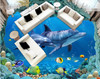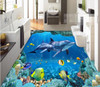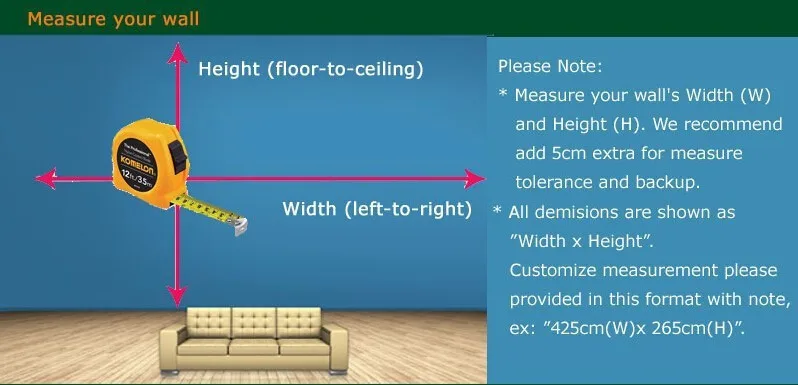# 3 d pvc flooring custom wall paper Underwater world --3d bathroom flooring picture mural photo wallpaper for walls 3d

(No reviews yet)
Condition:
New
Availability:
Imported
Options available
Shipping:
Calculated at Checkout
••••••MSRP: ₹7,899
₹3,909
— You save ₹3,990
Frequently bought together:

## Description

• Use:Living Room
• Charge Unit:Yuan/Roll
• Material:Other
• Style:Classic
• Function:Waterproof
• Function:Moisture-Proof
• Function:Mould-Proof
• Function:Smoke-Proof
• Function:Fireproof
• Function:Soundproof
• Function:Sound-Absorbing
• Function:Heat Insulation
• Function:Anti-static
• Textile Wallpaper Material:Non-Woven
• Usage:Commerce
• Usage:Entertainment
• Usage:Household
• Surface Treatment:Sandstone
• Pattern:Personalized Customization
• Type:Vinyl Wallpapers
• Flooring price: \$ / square meter.  It is not a roll.

1 square meter = 1pcs.   2 square meter=2pcs.   3 square meter =3pcs ...

Length * width = square meters = Order Quantity

you need place order is Length * width,

if the Length is 2m ,the width is 2m ,you need place order is 4 square meter

If you need to size is 4.1 to 4.5 square meters, you need to place an order for 4 square meters.

If you need to size is 4.6 to 4.9 square meters, you need to place an order for 5 square meters

Vinyl Wallpaper Type:Paper Back Vinyl Wallpapers

We have 12 fixed size order.

Quantity:1=140cm(L)×70.5cm(W) (4'3"×2'3”)

Quantity:2=200cm(L)×100cm(W) (6'5"×3'3”)

Quantity:3=208cm(L)×146cm(W) (6'8"×4'8”)

Quantity:4=200cm(L)×200cm(W) (6'5"×6'5”)

Quantity:5=250cm(L)×200cm(W) (7'6"×6'5”)

Quantity:6=300cm(L)×200cm(W) (9'8"×6'5”)

Quantity:7=300cm(L)×250cm(W) (9'8"×8'2”)

Quantity:8=400cm(L)×200cm(W) (13'6"×6'5”)

Quantity:9=350cm(L)×260cm(W) (11'4"×8'7”)

Quantity:10=360cm(L)×280cm(W) (11'8"×9'2”)

Quantity:12=400cm(L)×300cm(W) (13'6"×9'8”)

Quantity:16=500cm(L)×320cm(W) (16'4 "x 10'5"”)